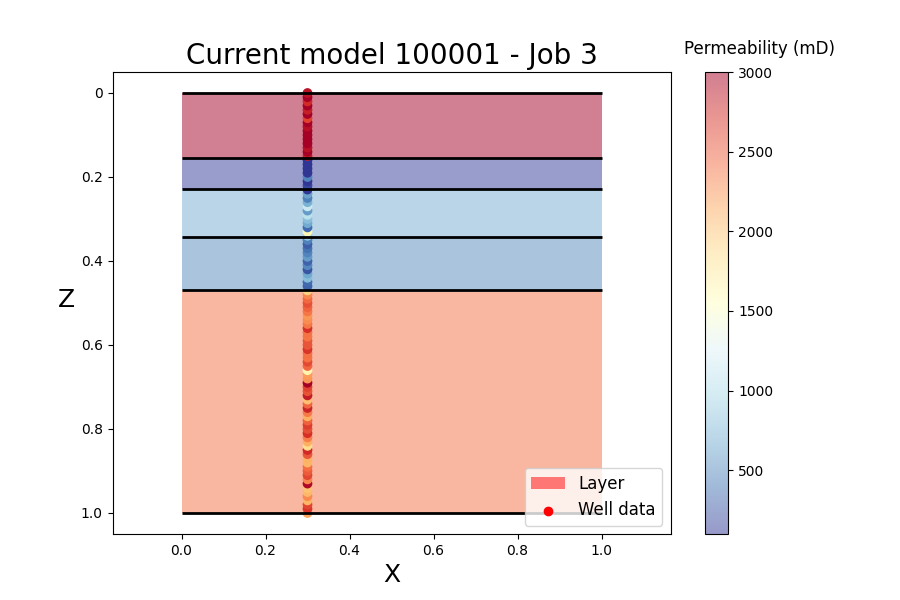Transdimensional inversion of flow data (TIFlow) is a library to perform transdimensional inversions in history matching problems. In contrast to Bayesian sequential modelling in which permeability values are inferred in a fixed reservoir geometry, TIFlow allows transdimensional Monte Carlo methods based on a reversible jump Markov chain Monte Carlo algorithm (RJMCMC). These approaches are a way to solve the inverse problem with a self-adaptive geological parameterization in which the number of model parameters is unknown.

The code considers a 2D layered reservoir model. The number of geological layers is variable and the algorithm will converge towards the appropriate reservoir parameter (permeability property) and reservoir geometry (number and location of layer interfaces), using flow data and static reservoir data.

The implemented RJMCMC algorithm is based on Thomas Bodin's work on transdimensional velocity model inversion in seismic tomography. Based on Bayes theorem and posterior probability sampling, a large number of models are generated and the outputs can be visualized by different figures.

Three inversion modes can be used, depending on the type of data chosen to constrain the model:

- Flow data can be used to perform history matching and sample the space model, but the problem is ill-posed and non-linear, so convergence may be difficult.

- More informative well log data of permeability can also be used as the only data constraint.

- Finally, it is possible to apply a joint inversion (sequential or not) of the two datasets to see the contribution of flow data to the problem and characterize the stratigraphic uncertainty of the reservoir model.

The use of dynamic reservoir data involves a flow simulation in the forward problem. This simulation is performed using RINGFlow code, developed by Mustapha Zakari. The model is currently a simple layer-cake model. In the future, it will be possible to use more complicated geometries (2D, 3D) based on the LUMOS remeshing method, allowing the insertion of implicit geological surfaces with complex shapes. The case of a fractured reservoir, where the number of fractures becomes unknown, is also possible.

The code is avalable on Github for sponsors here.

Main developper:

The code is developed by Julien Herrero in a Python library.

Related PhDs: